# Extension to bivariate density estimation

Outputs from the Grand Tour can be projections onto subspaces of any dimensionality, usually one two or three for convenience, as discussed in section. Extending the BKDE discussed above to more than one dimension is both natural and easy. A KDE is still specified in terms of some function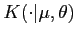and an estimate of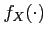based on the sample, at the point,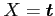is written as(27)

where(28)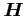is a bandwidth matrix and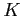is some bivariate kernel.

The estimate ofbased on the sample, at the point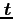,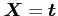is seen to again be of the form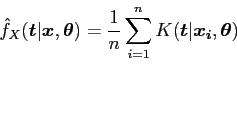(29)

where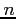is the number of data items. Note that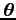may be considered to be a generalisation of bandwidth. Takingwith univariate data still leads to the standard KDE of (1), however, taking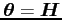leads to some higher dimensional estimate. In this case interest is in bivariate data and a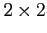matrix form of.

There are three possible orders of complexity for; if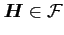, the class of all symmetric, positive, definitematrices, then there are 3 bandwidth parameters to choose; if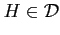, the subclass of all diagonal, positive, definitematrices, then there are 2 bandwidth parameters to choose; and finally, if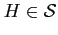, where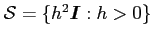, there is only 1 bandwidth parameter to choose.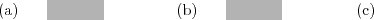However, a compromise between the work needed to estimate the bandwidth and the time taken to perform the estimation is required. Fukunaga (1972, p. 175) suggests a simple way of obtaining a bandwidth matrix of arbitrary orientation (see Silverman, 1986, p. 78). Taketo be of the form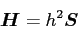(30)

where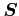is the covariance matrix. This approach is equivalent to sphering the data (i.e. transforming it to have unit covariance matrix).

This gives an estimate of the form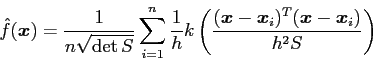(31)

It can be shown (Wand and Jones, 1995, p. 106) that, for the multivariate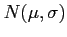distribution, the Asymptotic Mean Integrated Squared Error (AMISE) optimalsatisfies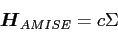(32)

for a scalar constant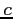. This implies that, for the multivariate Normal, sphering is appropriate. There is, unfortunately, no equivalent result for estimation of arbitrary density shapes. This is the approach taken for the version of the bivariate BKDE incorporated into the Grand Tour. By taking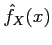to be a model for the data a likelihood function is constructed as before.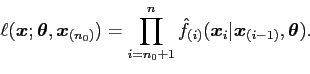(33)

Choice of prior foragain indicates belief in the smoothness of the underlying density and in the strength of that belief. This gives the posterior density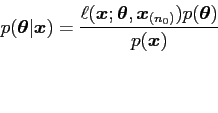(34)

and the predictive density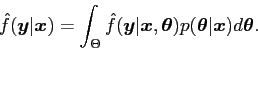(35)

danny 2009-07-23